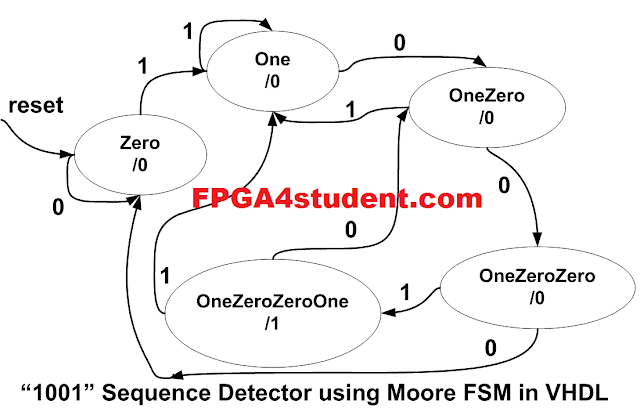# Full VHDL code for Moore FSM Sequence Detector

## This VHDL project presents a full VHDL code for Moore FSM Sequence Detector. A VHDL Testbench is also provided for simulation. The sequence to be detected is "1001".The Moore FSM state diagram for the sequence detector is shown in the following figure.### VHDL code for Moore FSM Sequence Detector is designed based on Moore FSM's state diagram and block diagram:

```-- fpga4student.com: FPGA projects, Verilog projects, VHDL projects
-- VHDL project: VHDL code for Sequence Detector using Moore FSM
-- The sequence being detected is "1001" or One Zero Zero One
library IEEE;
use IEEE.STD_LOGIC_1164.ALL;

entity VHDL_MOORE_FSM_Sequence_Detector is
port (
clock: in std_logic; --- clock signal
reset: in std_logic; -- reset input
sequence_in: in std_logic; -- binary sequence input
detector_out: out std_logic -- output of the VHDL sequence detector
);
end VHDL_MOORE_FSM_Sequence_Detector;

architecture Behavioral of VHDL_MOORE_FSM_Sequence_Detector is
type MOORE_FSM is (Zero, One, OneZero, OneZeroZero, OneZeroZeroOne);
signal current_state, next_state: MOORE_FSM;

begin
-- Sequential memory of the VHDL MOORE FSM Sequence Detector
process(clock,reset)
begin
if(reset='1') then
current_state <= Zero;
elsif(rising_edge(clock)) then
current_state <= next_state;
end if;
end process;
-- Next state logic of the VHDL MOORE FSM Sequence Detector
-- Combinational logic
process(current_state,sequence_in)
begin
case(current_state) is
when Zero =>
if(sequence_in='1') then
-- "1"
next_state <= One;
else
next_state <= Zero;
end if;
when One =>
if(sequence_in='0') then
-- "10"
next_state <= OneZero;
else
next_state <= One;
end if;
when OneZero =>
if(sequence_in='0') then
-- "100"
next_state <= OneZeroZero;
else
next_state <= One;
end if;
when OneZeroZero =>
if(sequence_in='1') then
-- "1001"
next_state <= OneZeroZeroOne;
else
next_state <= Zero;
end if;
when OneZeroZeroOne =>
if(sequence_in='1') then
next_state <= One;
else
next_state <= OneZero;
end if;
end case;
end process;
-- Output logic of the VHDL MOORE FSM Sequence Detector
process(current_state)
begin
case current_state is
when Zero =>
detector_out <= '0';
when One =>
detector_out <= '0';
when OneZero =>
detector_out <= '0';
when OneZeroZero =>
detector_out <= '0';
when OneZeroZeroOne =>
detector_out <= '1';
end case;
end process;
end Behavioral;```

### VHDL Testbench for Sequence Detector using Moore FSM:

```-- fpga4student.com: FPGA projects, Verilog projects, VHDL projects
-- VHDL project: VHDL code for Sequence Detector using Moore FSM
-- VHDL testbench for Moore FSM Sequence Detector
LIBRARY ieee;
USE ieee.std_logic_1164.ALL;

ENTITY tb_VHDL_Moore_FSM_Sequence_Detector IS
END tb_VHDL_Moore_FSM_Sequence_Detector;

ARCHITECTURE behavior OF tb_VHDL_Moore_FSM_Sequence_Detector IS

-- Component Declaration for the Moore FSM Sequence Detector in VHDL

COMPONENT VHDL_MOORE_FSM_Sequence_Detector
PORT(
clock : IN  std_logic;
reset : IN  std_logic;
sequence_in : IN  std_logic;
detector_out : OUT  std_logic
);
END COMPONENT;

--Inputs
signal clock : std_logic := '0';
signal reset : std_logic := '0';
signal sequence_in : std_logic := '0';

--Outputs
signal detector_out : std_logic;

-- Clock period definitions
constant clock_period : time := 10 ns;

BEGIN

-- Instantiate the Moore FSM Sequence Detector in VHDL
uut: VHDL_MOORE_FSM_Sequence_Detector PORT MAP (
clock => clock,
reset => reset,
sequence_in => sequence_in,
detector_out => detector_out
);

-- Clock process definitions
clock_process :process
begin
clock <= '0';
wait for clock_period/2;
clock <= '1';
wait for clock_period/2;
end process;

-- Stimulus process
stim_proc: process
begin
-- hold reset state for 100 ns.
sequence_in <= '0';
reset <= '1';
-- Wait 100 ns for global reset to finish
wait for 30 ns;
reset <= '0';
wait for 40 ns;
sequence_in <= '1';
wait for 10 ns;
sequence_in <= '0';
wait for 10 ns;
sequence_in <= '1';
wait for 20 ns;
sequence_in <= '0';
wait for 20 ns;
sequence_in <= '1';
wait for 20 ns;
sequence_in <= '0';
-- insert stimulus here
wait;
end process;

END;```

#### Simulation Waveform for Moore FSM Sequence Detector in VHDL:As shown in the simulation waveform of the VHDL Moore FSM sequence detector, the detector output only goes high when the sequence "1001" is detected.
Verilog code for Moore FSM Sequence Detector: here.